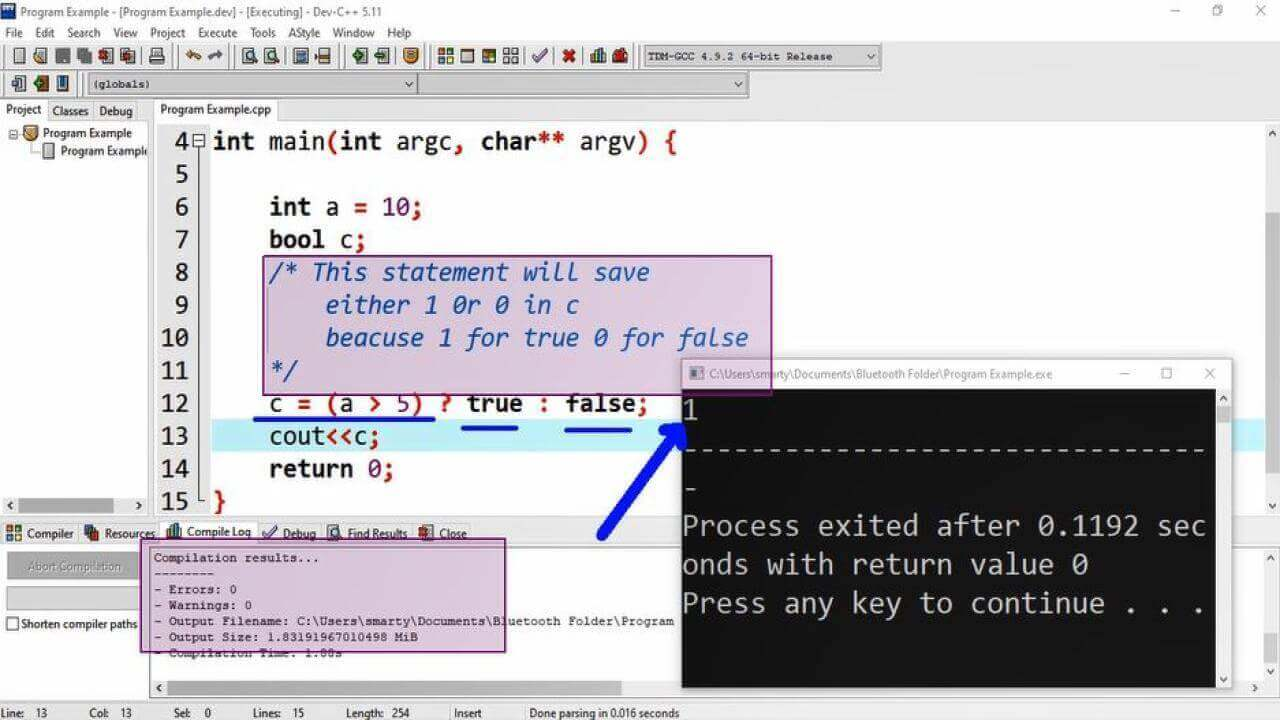# C++ Conditional operator

Conditional operator is most commonly used in place of if else statement. Similar to if else statement it also use relational or logical expression for specifying condition. It is also called Ternary operator. ? : symbols are used as conditional operator. Conditional operator can be used for two purposes.

• Execute some IO statements
• Assign value to variable

## Syntax:

### IO statement:

When condition will TRUE statement_1 will execute and statement_2 will execute when condition is FALSE.

( condition ) ? statement_1 : statement_2 ;

### Variable Assignment:

The value return by conditional operator will be assigned to the given variable. Here statement_1 and statement_2 may be some kind of computation.

variable = ( condition ) ? statement_1 : statement_2 ;

## Example:

### IO statement:

``````#include <iostream>
using namespace std;

int main(int argc, char** argv) {

int a = 1;

(a == 1) ? cout<<"Value is 1" : cout<<"Other Value";

return 0;
}``````

### Variable Assignment:

In this example first condition of conditional operator will evaluate, a it return TRUE so that 0 will be assigned to variable b.

``````#include <iostream>
using namespace std;

int main(int argc, char** argv) {

int a = 10;
int b;

b = (a > 5) ? 0 : 1;
cout<<b;

return 0;
}``````

## Output of Conditional Operator:## Conditional Operator with Multiple Statements:

We can also use block of statements in True or False case of ternary operator. For this purpose we have to close multiple statements in small parentheses separated by comma.

### Syntax:

(a < 20) ? (cout<<"True", cout<<" Here") : cout<<"False";

### Conditional operator example using multiple statements:

``````#include <iostream>
using namespace std;

int main()
{
int a = 10;
int b;
(a < 20) ? (cout<<"True", cout<<" Here") : cout<<"False";
return 0;
}``````